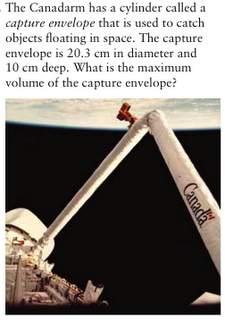## Wednesday, March 16, 2011

### Roemer's Volume Scribe Post

7.3Height = 10cm
Diameter = 20.3cm

r= d/2
r = 20.3 / 2
r = 10.5cm

v = π x r x r x h

v = 3.14 x 10.15 x 10.15

v = 323.49cm2
v = 323.49 x 10

v = 3234.9cm3

7.4Height = 10m
Diameter = 0.8 (inside) and 1 (outside)

Inside -
r = d/2
r = 0.8/2
r = 0.4

v = π x r x r x h
v = 3.14 x 0.4 x 0.4 x h
v = 0.5 x 10
v = 5m

Outside -
r = d/2
r = 1/2
r = 0.5

v = π x r x r x h
v = 3.14 x 0.5 x 0.5 x h
v = 0.78 x 10
v = 7.8m

I tried to look for the volume of the concrete to build the Culvert. I basically just took the volume from the inside, and subtracted it from the volume in the outside.

7.8 - 5m = 2.8m3